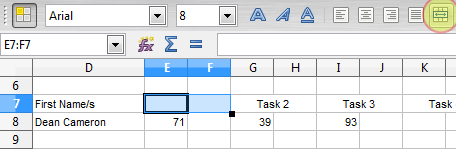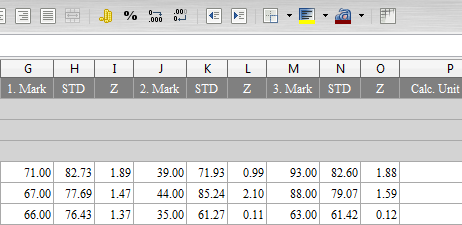# 08. Complex formulae

What we’re looking for in this section is to start filling out some more formulae and also set up the columns and rows a bit more:

Our spreadsheet is now getting a bit large to show in one small screenshot so from now on I will let you know if you can click on the smaller image to see the whole thing (like the example above).

There are four main alterations applied to our spreadsheet that we’re going to complete in this section, each of which are explained below.

1. First Name/s formula
The formula required for the “First Name/s” column is very similar to the “Surname” column. The only difference is that instead of starting at position 1 to extract a part of the name from “gv” we need to start a couple of characters after the comma. We also need to know where to stop extracting characters. Here’s a bit of a hint to get you started:

`=IF( ... , MID(gv.E5 , SEARCH( ... ) , 200) , "")`

Notice that the end position in our MID function is simply a very large arbitrary number (in our case 200). While you could probably go to the trouble of finding out exactly where to stop, the MID function will just retrieve all remaining characters if your end number is too large.

You should find the only things needing completion are the two areas where there is an ellipsis (an ellipsis is the three dots “…”), apart from this, the formula provided should be correct.

2. Cell Merging
There is a school of thought that you should avoid merging cells, however, I find it handy for keeping information organised and clarifying things on occasion. You may have noticed that Cells E7:F7 have been combined into one giant cell. This is done by selecting the cells you want to combine and then clicking the merge button (highlighted below):It will then combine the cells and centre any text you try to write in there. I’ve done this for E7:F7, G7:H7, and so on until we have room for five tasks.

3. Row Height and Column Width
While not a difficult thing to change, getting your cell sizes set up correctly is important. In order to change a row height or column width, simply right click on the letter or number representing the column or row respectively, then choose “Column Width…” or “Row Height…” (depending of course on whether you’re altering a row or column). In the dialog box that appears simply state the sizes you want.

The other thing you can do is move your mouse between two columns or rows and when the cursor changes from the regular white arrow to a black resize icon, you can left-click and resize a column or you can double left-click for the column to resize itself.

4. The Task formulae
And so I thought I’d leave the hardest bit till the end. This particular formula takes a bit of thought. The issue arises in that we don’t know how many tasks “gv” will contain. It could be anywhere from 0 – 5. So how can you tell if there is task data? Well, consider this snippet from the “gv” sheet we’ve started with:How do we as humans know how many tasks are in “gv“? Well, starting at Column G, we look to see if Cell G1 starts with the task number, then as we move 3 columns over we check to see if Cell J1 starts with the task number…when we get to Cell P1, it starts with the word “Calc“. Now that we know how to work out the number of tasks, how do we get our “Summary” sheet to do the same? Consider this formula for Cell E8:

`=IF(AND(MID(gv.G1,1,1)="1",gv.G5<>""),gv.G5,"")`

The only new function here is AND, but you can probably tell what it’s doing anyway…it allows you to check multiple things at once, in our case it’s checking to see if the first character in “gv.G1” is the number 1 AND it also checks to see if the contents of “gv.G5” are NOT blank.

That formula is probably one of the more complex ones we’re going to deal with. It involves turning some logical ideas into code that our spreadsheet can understand and so if you need to spend some time here getting it right and understanding it then do so. Once you understand it, get the formula in Cells G8, I8, K8 and M8 to do basically the same thing and then we’re ready to move on.